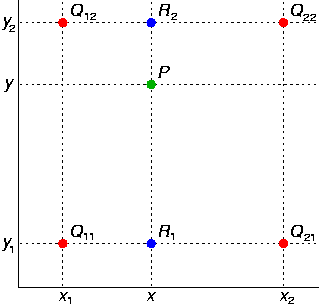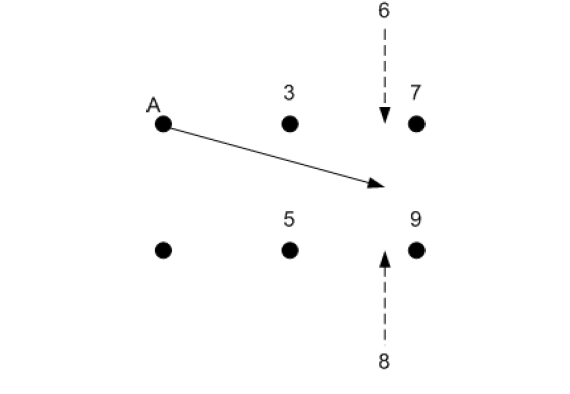## 前言

• 我们都知道，深度学习的成功的原因主要有两点：

1. 当前计算机的计算能力有很大提升；
2. 随着大数据时代的到来，当前的训练样本数目有很大的提升。
• 然而深度学习的一大问题是，有的问题并没有大量的训练数据，而由于深度神经网络具有非常强的学习能力，如果没有大量的训练数据，会造成过拟合，训练出的模型难以应用。因此对于一些没有足够样本数量的问题，可以通过已有的样本，对其进行变化，人工增加训练样本。

• 对于图像而言，常用的增加训练样本的方法主要有对图像进行旋转、移位等仿射变换，也可以使用镜像变换等等，这里介绍一种常用于字符样本上的变换方法，**弹性变换算法(Elastic Distortion)**。

• 该算法最先是由Patrice等人在2003年的ICDAR上发表的《Best Practices for Convolutional Neural Networks Applied to Visual Document Analysis》。本文主要是对论文中提出的弹性形变数据增强方法进行解读。

## 插播一下双线性插值的定义

• 双线性插值，顾名思义就是两个方向的线性插值加起来（这解释过于简单粗暴，哈哈）。所以只要了解什么是线性插值，分别在x轴和y轴都做一遍，就是双线性插值了。
• 线性插值：两个点A，B，要在AB中间插入一个点C（点C坐标在AB连线上），就直接让C的值落在AB的值的连线上就可以了。如A点坐标(0,0),值为3，B点坐标(0,2)，值为5，那要对坐标为(0,1)的点C进行插值，就让C落在AB线上，值为4就可以了。
• 但是如果C不在AB的线上肿么办捏，所以就有了双线性插值。如下图，已知Q12，Q22，Q11，Q21，但是要插值的点为P点，这就要用双线性插值了，首先在x轴方向上，对R1和R2两个点进行插值，这个很简单，然后根据R1和R2对P点进行插值，这就是所谓的双线性插值。## 1、仿射变换

• 仿射变换是最常用的空间坐标变换的方法之一，具体定义可参考冈萨雷斯的《数字图像处理第三版》50页。论文中是如下解释仿射变换的：
• 将仿射变换应用于图像，新像素的位置是由原始位置确定的，Δx(x,y)=1，Δy(x,y)=0代表向右移一个单位，Δx(x,y)= αx, Δy(x,y)= αy代表像素点由原点位置进行缩放。
• 上面说明了如何计算变换之后每个像素点的坐标，下图说明了如何应用位移字段来计算每个像素的新值（其实就是双线性插值的方法）：• 假设A是原点(0,0),而数字3,7,5,9是图像要转换的灰度等级，坐标分别为(1,0),(2,0),(1,-1),(1,-2),A的位移由Δx(0,0) = 1.75 and Δy(0,0) = -0.5给出，如箭头所示。通过评估原始图像的位置（1.75，-0.5）处的灰度级来计算新（扭曲）图像中的A的新灰度值。用于评估灰度级的简单算法是原始图像的像素值进行“线性插值”。尽管可以使用其他插值方案（例如，双三次和B样条插值），但双线性插值是最简单的插值方法之一，并且适用于以所选分辨率（29×29）生成附加的扭曲字符图像。
• 先水平插值，然后垂直插值，完成评估。箭头结束的位置在3,5,7,9的方格内，这样我们先计算箭头相对于它结束的方格的坐标。在这种情况下，它相对于正方形方格中的坐标是（0.75,0.5），假设该正方形的原点是左下角（也就是灰度值为5的点）。在此示例中，水平插值为：3 +0.75×(7-3)= 6;垂直插值为：8 +0.5×(6-8)= 7，因此A的新像素值为7.
• 对所有像素都进行了类似的计算。在给定图像之外的所有像素位置都假定有一个灰度值。

## 2、弹性形变

• 仿射变换改善了在MNIST数据集上的实验结果，但是实验在弹性形变后的数据集上取得了最好的结果。

### 那么什么是弹性形变呢？

• 首先创建随机位移场来使图像变形，即Δx(x,y) = rand(-1,+1)、Δy(x,y)=rand(-1,+1),其中rand(-1,+1)是生成一个在(-1,1)之间均匀分布的随机数，然后用标准差为σ的高斯函数对Δx和Δy进行卷积，如果σ值很大，则结果值很小，因为随机值平均为0.如果我们将位移场标准化（达到1的范数），则该字段接近常数，具有随机方向。
• 如果σ很小，则归一化后该字段看起来像一个完全随机的字段（如图2右上角所示）。
• 对于中间σ值，位移场看起来像弹性变形，其中σ是弹性系数。然后将位移场乘以控制变形强度的比例因子α。 在我们的MNIST实验（29x29输入图像）中，产生最佳结果的值是σ = 4和α= 34。
• 将经过高斯卷积的位移场乘以控制变形强度的比例因子α，得到一个弹性形变的位移场，最后将这个位移场作用在仿射变换之后的图像上，得到最终弹性形变增强的数据。作用的过程相当于在仿射图像上插值的过程，最后返回插值之后的结果。
• 关于高斯卷积的原理可以参考这篇文章：高斯卷积滤波
• 如果文章看完文章，还是不太懂弹性形变数据增强的原理的话，可以结合代码一起看，下面是参考代码，我都有注释。

## 3、参考代码

# -*- coding:utf-8 -*-"""@author:TanQingBo@file:elastic_transform.py@time:2018/10/1221:56"""# Import stuffimport osimport numpy as npimport pandas as pdimport cv2from scipy.ndimage.interpolation import map_coordinatesfrom scipy.ndimage.filters import gaussian_filterimport matplotlib.pyplot as plt		# Function to distort image  alpha = im_merge.shape*2、sigma=im_merge.shape*0.08、alpha_affine=sigmadef elastic_transform(image, alpha, sigma, alpha_affine, random_state=None):    """Elastic deformation of images as described in [Simard2003]_ (with modifications).    .. [Simard2003] Simard, Steinkraus and Platt, "Best Practices for         Convolutional Neural Networks applied to Visual Document Analysis", in         Proc. of the International Conference on Document Analysis and         Recognition, 2003.				     Based on https://gist.github.com/erniejunior/601cdf56d2b424757de5    """    if random_state is None:        random_state = np.random.RandomState(None)    shape = image.shape    shape_size = shape[:2]   #(512,512)表示图像的尺寸				    # Random affine    center_square = np.float32(shape_size) // 2    square_size = min(shape_size) // 3    # pts1为变换前的坐标，pts2为变换后的坐标，范围为什么是center_square+-square_size？    # 其中center_square是图像的中心，square_size=512//3=170    pts1 = np.float32([center_square + square_size, [center_square + square_size, center_square - square_size],                       center_square - square_size])    pts2 = pts1 + random_state.uniform(-alpha_affine, alpha_affine, size=pts1.shape).astype(np.float32)    # Mat getAffineTransform(InputArray src, InputArray dst)  src表示输入的三个点，dst表示输出的三个点，获取变换矩阵M    M = cv2.getAffineTransform(pts1, pts2)  #获取变换矩阵    #默认使用 双线性插值，    image = cv2.warpAffine(image, M, shape_size[::-1], borderMode=cv2.BORDER_REFLECT_101)    # # random_state.rand(*shape) 会产生一个和 shape 一样打的服从[0,1]均匀分布的矩阵    # * 2 - 1 是为了将分布平移到 [-1, 1] 的区间    # 对random_state.rand(*shape)做高斯卷积，没有对图像做高斯卷积，为什么？因为论文上这样操作的    # 高斯卷积原理可参考：https://blog.csdn.net/sunmc1204953974/article/details/50634652    # 实际上 dx 和 dy 就是在计算论文中弹性变换的那三步：产生一个随机的位移，将卷积核作用在上面，用 alpha 决定尺度的大小    dx = gaussian_filter((random_state.rand(*shape) * 2 - 1), sigma) * alpha    dy = gaussian_filter((random_state.rand(*shape) * 2 - 1), sigma) * alpha    dz = np.zeros_like(dx)  #构造一个尺寸与dx相同的O矩阵				    # np.meshgrid 生成网格点坐标矩阵，并在生成的网格点坐标矩阵上加上刚刚的到的dx dy    x, y, z = np.meshgrid(np.arange(shape), np.arange(shape), np.arange(shape))  #网格采样点函数    indices = np.reshape(y + dy, (-1, 1)), np.reshape(x + dx, (-1, 1)), np.reshape(z, (-1, 1))    # indices = np.reshape(y+dy, (-1, 1)), np.reshape(x+dx, (-1, 1)), np.reshape(z, (-1, 1))				    return map_coordinates(image, indices, order=1, mode='reflect').reshape(shape)				# Define function to draw a griddef draw_grid(im, grid_size):    # Draw grid lines    for i in range(0, im.shape, grid_size):        cv2.line(im, (i, 0), (i, im.shape), color=(255,))    for j in range(0, im.shape, grid_size):        cv2.line(im, (0, j), (im.shape, j), color=(255,))				if __name__ == '__main__':    img_path = 'E:/liverdata/nii/png/img'    mask_path = 'E:/liverdata/nii/png/label'    # img_path =  '/home/changzhang/ liubo_workspace/tmp_for_test/img'    # mask_path = '/home/changzhang/liubo_workspace/tmp_for_test/mask'				    img_list = sorted(os.listdir(img_path))    mask_list = sorted(os.listdir(mask_path))    print(img_list)				    img_num = len(img_list)    mask_num = len(mask_list)				    assert img_num == mask_num, 'img nuimber is not equal to mask num.'				    count_total = 0    for i in range(img_num):        print(os.path.join(img_path, img_list[i]))   #将路径和文件名合成一个整体        im = cv2.imread(os.path.join(img_path, img_list[i]), -1)        im_mask = cv2.imread(os.path.join(mask_path, mask_list[i]), -1)				        # # Draw grid lines        # draw_grid(im, 50)        # draw_grid(im_mask, 50)				        # Merge images into separete channels (shape will be (cols, rols, 2))        im_merge = np.concatenate((im[..., None], im_mask[..., None]), axis=2)				        # get img and mask shortname        (img_shotname, img_extension) = os.path.splitext(img_list[i])  #将文件名和扩展名分开        (mask_shotname, mask_extension) = os.path.splitext(mask_list[i])				        # Elastic deformation 10 times        count = 0				        while count < 10:            # Apply transformation on image  im_merge.shape表示图像中像素点的个数            im_merge_t = elastic_transform(im_merge, im_merge.shape * 2, im_merge.shape * 0.08,                                           im_merge.shape * 0.08)            # Split image and mask            im_t = im_merge_t[..., 0]            im_mask_t = im_merge_t[..., 1]				            # save the new imgs and masks            cv2.imwrite(os.path.join(img_path, img_shotname + '-' + str(count) + img_extension), im_t)            cv2.imwrite(os.path.join(mask_path, mask_shotname + '-' + str(count) + mask_extension), im_mask_t)				            count += 1            count_total += 1        if count_total % 100 == 0:            print('Elastic deformation generated {} imgs', format(count_total))            # # Display result            # print 'Display result'            # plt.figure(figsize = (16,14))            # plt.imshow(np.c_[np.r_[im, im_mask], np.r_[im_t, im_mask_t]], cmap='gray')            # plt.show()